## Topic : introduction to estimation

Class : jss 2

Subject : Maths

Objectives : At the end of the lesson, the  students should be able to

a. Define estimation

b. State simple rules of estimation

## What is Estimation?

To estimate means to find something close to the correct answer. In other words, you are approximating. For example, the American statistic for the ideal number of children is 2.5. Now, obviously, nobody can have half a kid. We can estimate this exact statistic to either 2 or 3 children. To write estimate, we use the squiggly equal sign. Estimation can help you in various circumstances both in math and in real life.

## General Rule of Estimating

When it comes to estimating in math, there is a general rule for you to follow. This general rule tells you to look at the digit to the right of the digit you want to estimate, and if it is less than 5 then you round down, and if it is greater than 5, you round up.

So, for example, if you wanted to round to the nearest whole number here, you would look at the digit right after the decimal since that is the digit to the right of the digit you want to round.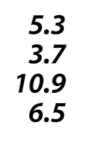You can think to yourself, ‘If I want to round to this place, what is the digit that is to the right of that?’ For whole numbers, think what is the digit to the right of the whole number? It’s the digit right after the decimal.

If we want to round these numbers to the nearest whole number, we’ll need to look at the digit right after the decimal. Our general rule tells us that if our digit that is to the right of the digit we want to estimate is under 5, then we round down, and if it’s greater, then we round up. Okay, that sounds easy enough.

Let’s start rounding: 5.3 becomes 5; 3.7 becomes 4; 10.9 becomes 11. Oh wait. What about the 6.5? It’s right in the middle. What do I do? For estimating purposes, whenever you see a 5, you will round up.

When you round down, you round down to your nearest number. When it came to rounding 5.3, I rounded it down to 5 because that was the closest one. I can draw it on the number line like this, and I can plainly see that 5 is the closest whole number to 5.3.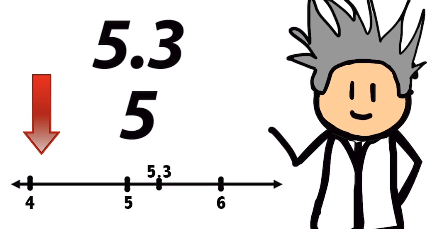So rounding down doesn’t mean going down one whole number, it just means going to the closest whole number which, when it comes to rounding down, means you keep the same whole number that you see. Rounding up means you go up to the next number. Like when I rounded 10.9 to 11. 11 is the closest whole number to 10.9. And, you can see it easily if you draw it out on a number line.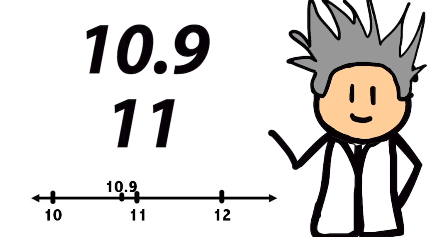## Estimating to Different Places

You can estimate to whatever place you want. Let’s say you wanted to estimate to the nearest tens place. This means instead of estimating to the nearest whole number, you estimate to the digit that is in the tens place or the second one to the left of the decimal. To estimate this digit, you would look at the digit in the ones place because that is the digit to the right of the digit you want to estimate.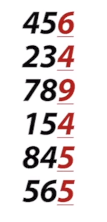Estimating these numbers to the tens place, I would follow the same general rule as before, when we were rounding with decimals. Here, 456 becomes 460; 234 becomes 230; 789 becomes 790; 154 becomes 150; 845 becomes 850, and 565 becomes 560. Noticed how when I see a 5, I alternate rounding up and down? That is why 845 becomes 850, but 565 does not become 570. With all the others I followed the rule and did what I did when rounding to the nearest whole number.

What do you think we would do if we wanted to estimate to the hundreds place? Which digit would we look at?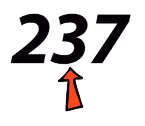237 can be estimated to the nearest hundred as 200.